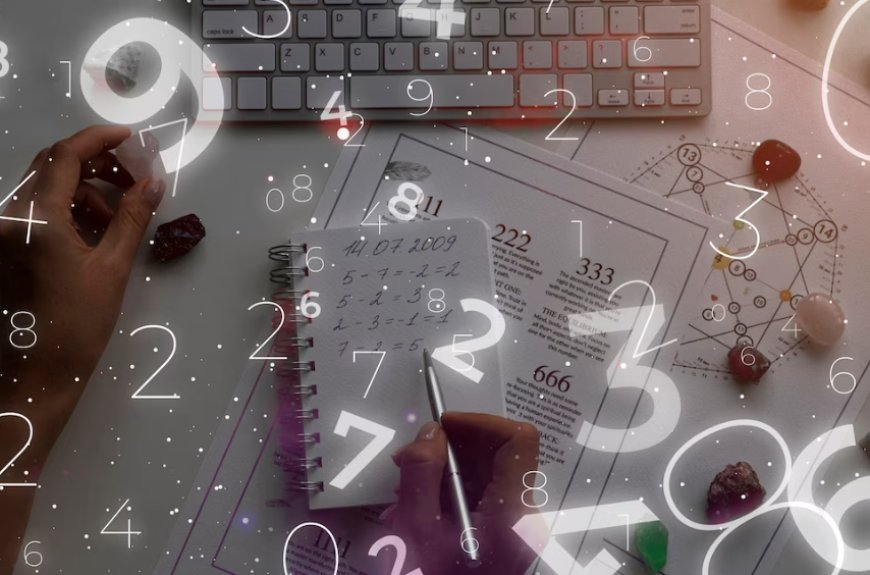# Mathematics for Machine Learning

## Mathematics for Machine Learning: The essential framework that powers algorithms, enabling computers to learn, predict, and make intelligent decisions.

Mar 13, 2019 - 13:09
Jun 19, 2023 - 11:43
0  123Past 4 years, I have been actively involved in coaching Machine Learning (ML) aspirants and trained more than 5000 in-person in the field of Machine Learning and Artificial Intelligence. One of the frequently asked questions is about the level of Mathematics required for Machine Learning.

## First, Is Mathematics really required for applying Machine Learning to solve business problems?

Well, Mathematics is a core domain in the field of Machine Learning but for applied ML, it is NOT required to have a good understanding of the mathematics behind it. There are many high-level software packages for Machine Learning such as Python Sklearn from scikit-learn, TensorFlow from Google, etc., which abstracts the mathematically modeling part and exposes a simple interface for implementing ML models for solving practical business problems. Having said that, gaining a sound knowledge of Mathematics related to ML, enables an intuitive understanding of the internal mechanics of ML algorithms and significantly improves the ability to fine-tune the models for better results.

## What are the areas in Mathematics relevant to Machine Learning?

Mathematics is a vast domain, but fortunately, only a few areas are related to Machine Learning. They are as below
1. Statistics: Deals with collecting, analyzing data, hypothesis, data distributions, etc.,
2. Linear Algebra: Linear Transformations, Matrices
3. Probability
4. Calculus: Derivates

## Where to start learning mathematics knowledge for Machine Learning?

There are a plethora of articles and books on mathematics, it would take significant time to understand where to start. I recommend NOT to start with a detailed theory as learning without understanding the practical application like where a math concept is used in ML gets boring.

A quick glance at the below topics is a good start to move to the next phase of learning through examples of practical application in ML.

1. Number Theory (Integers, Fractions, Decimals, Ratio and Proportions, Percentage, Exponents and Roots, Real Numbers, Sequence and Series)
2. Sets Theory (Sets and their Representations, Equal Sets and Subsets, Power Set and Universal Set, Operations on Sets, Complement of a Set)
3. Algebra (Algebraic Expressions, Linear and Quadratic equations, Linear and Quadratic Inequalities, Algebraic functions, Logarithms, Binomial Theorem, Vector Algebra)
4. Matrices (Matrix Introduction, Types and Operations of Matrices, Transpose of a Matrix, Symmetric and Skew Symmetric Matrices, Transformation of Matrix, Invertible Matrices, Determinant and it’s Properties, Matrix Solution for Area of a Triangle, Minors, Cofactors, Adjoint and Inverse of a Matrix, Determinants and Matrix Applications)
5. Probability (Counting Methods, Permutations, Factorials, Combinations,etc.,)
6. Calculus (Introduction and Intuition behind Derivatives, Limits, and Derivatives, Limits of Functions, Continuity, and Differentiability, Derivatives Applications, Differential Equations, Chain Rule, Partial Derivatives and more..)

The above concepts can be learned through maths books, articles/blogs, and YouTube videos. I recommend YouTube channel @Khanacademy. Mr. Sal Khan does an amazing job of presenting math concepts in an easy and understandable way that helps you to learn and gain confidence without getting bored. If you like Khan’s videos then it could be your starting point to learn essential mathematics for ML. Once you gained fairly good understanding, then you need to head towards mathematical theories, which are used in modeling Machine Learning algorithms.

I would be writing a series of blogs on Machine Learning algorithms, which also covers related Mathematical concepts such as Lagrange’s theorem, Hessian matrix, Taylor’s theorem, etc., but as I mentioned at the beginning of the article, you need NOT dwell into details of proofs of these concepts rather understand conclusions, which are required to understand maths beyond ML algorithms.

It is highly recommended to get hands-on with coding while learning Mathematics. There are many popular Python packages such as Scipy.org, Numpy, TensorFlow, etc., to practice math concepts. I suggest to explore and learn more about this.